# Short Answers - Atoms and Molecules, Science, Class 9 Class 9 Notes | EduRev

## Class 9 : Short Answers - Atoms and Molecules, Science, Class 9 Class 9 Notes | EduRev

The document Short Answers - Atoms and Molecules, Science, Class 9 Class 9 Notes | EduRev is a part of the Class 9 Course Class 9 Science by VP Classes.
All you need of Class 9 at this link: Class 9

Q1. Give the unit to measure size of atom and give size of hydrogen atom.
Ans.
The unit to measure size of atom is nanometer, size of hydrogen atom is 10−10 m.

Q2. What is IUPAC, give its one function?
Ans
. IUPAC stands for International Union of Pure and Applied Chemistry. It approves the  names of elements.

Q3. Give the Latin name for sodium, potassium, gold and mercury.
Ans.
Sodium → Natrium, Gold → Aurum Potassium → Kalium, Mercury → Hydrargyrum

Q4. What is the ratio by mass of combining elements in H2O, CO2 and NH3?
Ans.
H2O ratio by mass of combining elements 2 : 16 → 1 : 8 (H : O)
CO2 ratio by mass of combining elements 12 : 32 → 3 : 8 (C : O)
NH3 ratio by mass of combining elements 14 : 3 → 14 : 3 (N : H)

Q5. Define valency and give the valency for the following elements: Magnesium, Aluminium, Chlorine and Copper.
Ans.
Valency: The combining capacity of an element is called its valency.
Valency of the following elements:
Magnesium – 2
Aluminium – 3
Chlorine – 1
Copper – 2

Q6. What is polyatomic ion? Give one example.
Ans.
A group of atoms carrying a charge is known as a polyatomic ion.
E.g., Ammonium – NH4+
Nitrate – NO3−

Q7. Write down the formula for: Copper nitrate, calcium sulphate and aluminium hydroxide.
Ans.
Chemical formula: Copper nitrate → Cu(NO3) Calcium sulphate → CaSO4 Aluminium hydroxide → Al(OH)3

Q8. What is formula unit mass? How is it different from molecular mass?
Ans.
The formula unit mass of a substance is a sum of the atomic masses of all  atoms in a formula unit of a compound. The constituent particles of formula unit mass are ions and the constituent particles of molecular mass are atoms.

Q9. Find the number of moles in the following: (i) 50 g of H2O (ii) 7 g of Na
Ans.
Number of moles in
(i) Molar mass of H2O = 18 g Given mass of H2O = 50 g
∴ No. of moles in 50 g of H2O = (50/18)= 2.78 moles.

(ii) Molar mass of Na = 23 g Given mass of Na = 7 g
∴ No. of moles in 7 g of Na = (7/23)= 0.304 moles.

Q10. Find the number of atoms in the following:
(i) 0.5 mole of C atom (ii) 2 mole of N atom
Ans.
(i) 0.5 mole of C atom:
Number of atoms in 1 mole of C atom = 6.022 × 1023 atoms
Number of atoms in 0.5 mole of C atom = 6.022 × 1023 × 0.5 = 3.011 × 1023 atoms

(ii) 2 mole of N atom:
Number of atoms in 1 mole of N atom = 6.022 × 1023 atoms
∴ Number of atoms in 2 mole of N atom = 6.022 × 2 × 1023 = 1.2044 × 1024 atoms

Q11. Find the mass of the following:
(i) 6.022 × 1023 number of O2 molecules
(ii) 1.5 mole of CO2 molecule
Ans.
(i) 6.022 × 1023 number of O2 molecules:
Mass of 1 mole of O2 molecule = 6.022 × 1023 molecules = 32g

(ii) 1.5 mole of CO2 molecule:
Mass of 1 mole of CO2 molecule = 6.022 × 1023 molecules = 44g
Mass of 1.5 mole CO2 molecule = 44 × 1.5 = 66g

Q12. Show the relationship between mole, Avogadro number and mass.
Ans.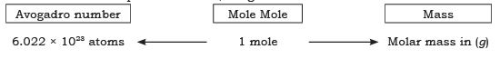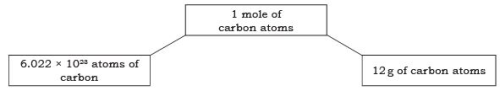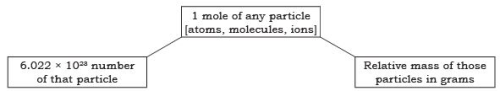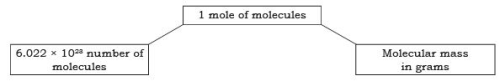Q13. What are the rules for writing the symbol of an element?
Ans.
IUPAC → International Union of Pure and Applied Chemistry approves name of elements.
Symbols are the first one or two letters of the element’s name in English. The first letter of a symbol is always written as a capital letter (upper case) and the second letter as a small letter (lower case).
e.g., Hydrogen →  H
Helium → He
Some symbols are taken from the names of elements in Latin, German or Greek. e.g., Symbol of iron is Fe, its Latin name is Ferrum.
Symbol of sodium is Na, its Latin name is Natrium.

Q14. Explain relative atomic mass and relative molecular mass.
Ans.
Relative atomic mass: It can be defined as the number of times one atom of given element is heavier than (1/12) th of the mass of an atom of carbon-12.
Relative Molecular Mass: It is defined as the number of times one molecule of a substance or given element is heavier than (1/12) th of the mass of one atom of carbon-12.

Q15. The formula of carbon-dioxide is CO2. What information do you get from this formula?
Ans.
(i) CO2 represents carbon-dioxide.
(ii) COis one molecule of carbon-dioxide.
(iii) COis one mole of carbon-dioxide i.e., it contains 6.022 × 1023 molecules of carbon dioxide.
(iv) CO2 contains 1 atom of carbon and two atoms of oxygen.
(v) CO2 represents 44 g of molar mass.

Q16. State 3 points of difference between an atom and an ion.
Ans.

 Atom Ion 1. An atom has no charge. An ion has either positive or negative charge. 2. Number of electrons = number of protons. Number of electrons ≠ number of protons. 3. Atom is reactive. Ion is stable.

Q17. Calculate the formula unit mass of NaCl and CaCl2. (Na = 23, Cl = 35.5, Ca = 40)
Ans.
Formula unit mass of NaCl = 23 + 35.5
= 58.5 u
Formula unit mass of CaCl2 = 40 + (2 × 35.5)
= 40 + 71
= 111 u

Q18. Write down the chemical formula for the following compounds:
(a) Aluminium carbonate
(b) Calcium sulphide
(c) Zinc carbonate
(d) Copper phosphate
(e) Magnesium bicarbonate
(f) Aluminium hydroxide.
Ans.
The chemical formula are: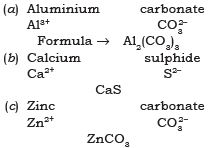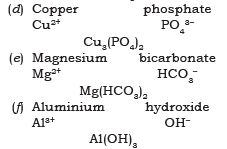Q19. The ratio by mass for hydrogen and oxygen in water is given as 1 : 8 respectively. Calculate the ratio by number of atoms for a water molecule.
Ans.
The ratio by number of atoms for a water molecule are: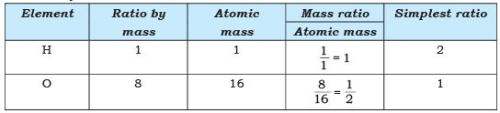Thus, the ratio by number of atoms for water is H : O = 2 : 1.

Q20. Give the atomicity of the following compounds:
(a) Ca(OH)
(b) Mg(HCO3)
(c) Cu2O.
(d) H2SO4
(e) Al2(SO4)3
(f) MgCl
Ans.
The atomicity of the molecules are:
(a) Ca(OH)2 → 05
(b) Mg(HCO3)2 → 11
(c) Cu2O → 03
(d) H2SO→ 07
(e) Al2(SO4)3 → 17
(f) MgCl→ 03

Q21. Explain the difference between 2O, O2 and O3.
Ans.
2O → It represents 2 atoms of oxygen (cannot exist independently).
O2 → It represents one molecule of oxygen (made up of 2 atom) can exist freely.
O3 → It represents one molecule of ozone (made up of 3 atoms) it can exist independently.

Q22. 1.50 g sample of barium hydroxide was dissolved in water. The total volume of the solution was 100 cm3.
A 25.0 cm3 portion of the barium hydroxide solution was titrated against hydrochloric acid. The volume of hydrochloric acid required was 18.75 cm3.
Ba(OH)+ 2HCl → BaCl2 + 2H2O

(i) Calculate how many moles of barium hydroxide were in the 25.0 cm3 portion used in the titration.

(ii) Calculate the concentration of the hydrochloric acid used.
Ans
. (i) Moles of barium hydroxide = 0.00219 mol. (ii) Moles of HCl = 2 × 0.00219 = 0.00438.

Q23. Analysis of a certain compound showed that 39.348 grams of it contained 0.883 grams of hydrogen, 10.497 grams of Carbon, and 27.968 grams of Oxygen. Calculate the empirical formula of the compound.
Ans.

• First divide the amount by the atomic mass to get the number of moles of each kind of atom in the formula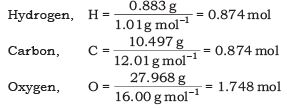•  Analysis of the ratio shows that the first two are identical and that the third is twice the other two. Therefore the ratio of H to C to O is 1 to 1 to 2. The empirical formula is HCO2.

Q24. If the molecular mass of the above compound HCOis 90.0. Calculate the molecular formula.
Ans.

• The empirical formula mass of HCO2 is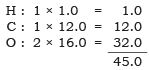•  Note that 45 is exactly half of the molecular mass of 90. So the formula mass of HCO2 is exactly half of the molecular mass. Hence the molecular formula is double that of the empirical formula i.e., H2C2O4.

Q25. 2K3PO+ 3BaCl→ Ba3(PO4)2 + 6KCl Calculate the mass of BaCl2 that is required to react with 21.2 grams of K3PO4 in the above reaction.
Ans.
Given molar mass of K3PO= 3(39.1) + (31.0) + 4(16.0) = 212.1 g mol–1 Required molar mass of BaCl2 = 137.3 + 2(35.5) = 208.3 g mol–1 = (21.2 g K3PO4)(3 mol BaCl2)(208.3 g mol–1 BaCl2) = 31.2 g BaCl2.

Q26. Zinc reacts with copper sulfate in a single replacement reaction as follows Zn (s) + CuSO4 (aq) → ZnSO(aq) + Cu (s) 50.00 grams of zinc metal was added to excess copper sulphate dissolved in a water solution. 42.50 grams of copper was recovered. Calculate the theoretical yield of copper in this experiment
(i) Solve the mass-mass Stoichiometry problem to find the theoretical yield.
(ii) Find the percent yield.
Ans
. (i) Mass Cu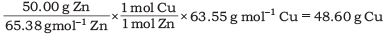(ii) Percent Yield of Cu =Q27. Silver can be produced by reacting silver nitrate with magnesium in the following reaction Mg(s) + 2AgNO3 (aq) → Mg(NO3)2 (aq) + 2Ag (s) How much Silver can be recovered by reacting a silver nitrate solution with 50.00 grams of powdered magnesium? Assume that 95% of the silver can be recovered.
(i) Solve the mass-mass Stoichiometry problem to find the theoretical yield.
(ii) Find the percent yield.
Ans.
(i) Mass Ag =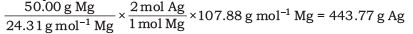(ii) Percent yield of Ag = 443.77 g Ag × 0.95 = 421.58 g

Q28. Compound X has the following percentage composition by mass: C = 26.7%, O = 71.1% and H = 2.2%. Calculate the empirical formula of compound B.
Ans.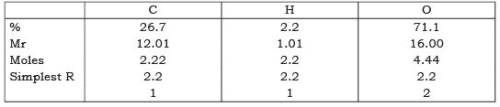Empirical formula: CHO2.

Q29. Work out the molecular formula for each of the following given the empirical formula and the relative molecular mass:
(a) CH2, Mr = 70 (b) OH, Mr = 34 (c) C2H5O, Mr = 90
Ans.
(a) CH2, Mr = 70(12.01) + (2 × 1.01) = 14.03
70 ÷ 14.03 = 5
CH× 5 = C5H10

(b) OH, Mr = 34 (16.00) + (1.01) = 17.01
34 ÷ 17.01 = 2
OH × 2 = H2O2

(c) C2H5O, Mr = 90 (2 × 12.01) + (5 × 1.01) + (16.00) = 45.07
90 ÷ 45.07 = 2
C2H5O × 2 = C4H10O2

Offer running on EduRev: Apply code STAYHOME200 to get INR 200 off on our premium plan EduRev Infinity!

75 docs|14 tests

,

,

,

,

,

,

,

,

,

,

,

,

,

,

,

,

,

,

,

,

,

,

,

,

,

,

,

;